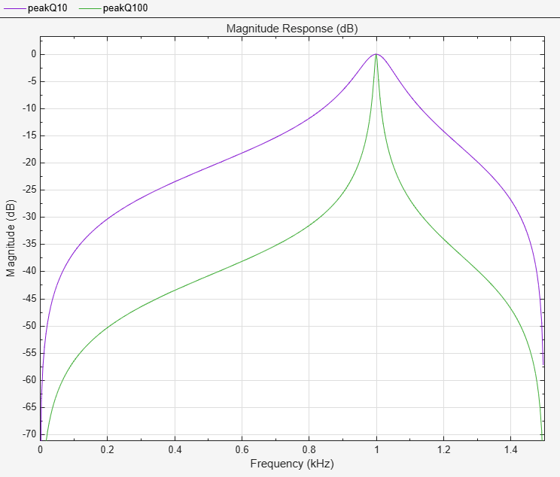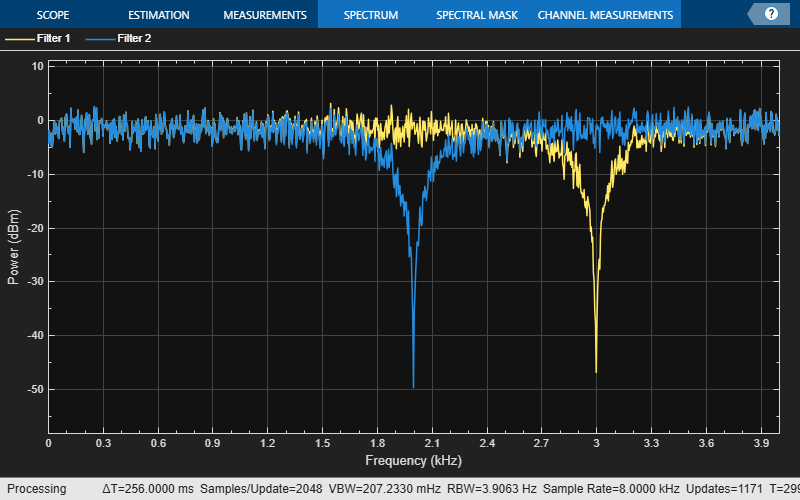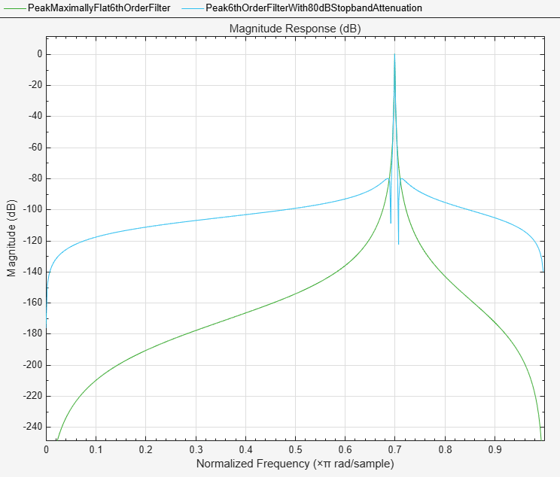# Design of Peaking and Notching Filters

This example shows how to design peaking and notching filters. Filters that peak or notch at a certain frequency retain or eliminate a particular frequency component of a signal. The design parameters for the filter are the frequency at which the peak or notch is desired, and either the 3-dB bandwidth or the filter's Q factor. Moreover, given these specifications, by increasing the filter order, it is possible to obtain designs that more closely approximate an ideal filter.

### Second Order Notch Filters

Suppose you need to eliminate a 60 Hz interference in a signal sampled at 3000 Hz. A notch filter can be used for such a purpose. The `iirnotch` function can be used to compute the coefficients of a second order notch filter.

Here is an example:

```F0 = 60; % Interference is at 60 Hz Fs = 3000; % Sampling frequency is 3000 Hz BW = 6; % Choose a bandwidth factor of 6Hz [num1,den1] = iirnotch(F0/(Fs/2),BW/(Fs/2)); fvtool(num1,den1,'Fs',Fs,'Color','white');```An equivalent way of designing the filter is to specify the quality factor and obtain the 3 dB bandwidth. Quality factor is defined as the ratio of the notch or peak frequency ${\mathit{F}}_{0}$ and the 3 dB bandwidth $\mathit{BW}$. Mathematically, Q factor is given by $Q={\mathit{F}}_{0}/\mathit{BW}$. In the above case, the value of the quality factor is 10. Specifying the bandwidth is a more convenient way of achieving exactly the desired shape for the designed filter. Ther Q factor of the filter is a measure of how well the desired frequency is isolated from other frequencies. For a fixed filter order, a higher Q factor is accomplished by pushing the poles closer to the zeros.

Visualize the magnitude response of the filter using `fvtool`.

```Q2 = 100; % Choose a Q factor of 100 [num2,den2] = iirnotch(F0/(Fs/2),F0/(Q2*Fs/2)); fvt = fvtool(num1,den1,num2,den2,'Fs',Fs,'Color','white'); legend(fvt,'Q = 10','Q = 100');```### Second order Peak Filters

Peaking filters are used to retain only a single frequency component (or a small band of frequencies) from a signal. The `iirpeak` function can be used to compute the coefficients of a second order peak filter.

```F0 = 1000; % Interference is at 60 Hz Fs = 3000; % Sampling frequency is 3000 Hz Q1 = 10; [num1,den1] = iirpeak(F0/(Fs/2),F0/(Q1*Fs/2)); Q2 = 100; [num2,den2] = iirpeak(F0/(Fs/2),F0/(Q2*Fs/2)); fvt = fvtool(num1,den1,num2,den2,'Fs',Fs,'Color','white'); legend(fvt,'Q = 10','Q = 100');```### Time varying Second Order Notch filter Implementation

Using time-varying filters requires changing the coefficients of the filter while the simulation runs. The DSP System Toolbox™ provides certain features such as the` iirnotch` function and the `dsp.NotchPeakFilter` object to design time-varying tunable notch filters. These features compute the filter coefficients directly.

#### Dynamic simulation with static filter

In order to implement a time varying filter, create a dynamic setup to simulate the filter and implement the filter with time-varying design parameters.

Start by creating a dynamic (streamed) simulation with filters whose coefficients do not change. Create two second-order notch filters, one using the `dsp.SOSFilter` object and the second using the `dsp.NotchFilter` object. In the first filter, set the center frequency to 1 kHz, and the bandwidth at -3 dB to 500 Hz. Calculate the coefficients of this filter directly using the `iirnotch` function. In the second filter, set the center frequency to 3 kHz and the bandwidth at -3 dB to 500 Hz. The sample rate for both filters is 8 kHz.

```Fs = 8e3; % 8 kHz sampling frequency F01 = 1e3; % Notch at 1 kHz for Filter 1 BW = 500; % 500 Hz bandwidth for both filters [b, a] = iirnotch(F01/(Fs/2), BW/(Fs/2)) % Filter 1 coefficients```
```b = 1×3 0.8341 -1.1796 0.8341 ```
```a = 1×3 1.0000 -1.1796 0.6682 ```
```sosFilter = dsp.SOSFilter(b,a); F02 = 3e3; % Notch at 3 kHz for Filter 2 npFilter = dsp.NotchPeakFilter('CenterFrequency',F02,'Bandwidth',BW,... 'SampleRate',Fs); scope = spectrumAnalyzer('PlotAsTwoSidedSpectrum', false, ... 'SampleRate', Fs, ... 'AveragingMethod','exponential',... 'ForgettingFactor',.95,... 'ChannelNames',{'Filter 1','Filter 2'},... 'ShowLegend',true); samplesPerFrame = 256; nFrames = 8192; for k = 1:nFrames x = randn(samplesPerFrame, 1); y1 = sosFilter(x); y2 = npFilter(x); scope([y1,y2]); end```#### Dynamic simulation with time-varying filter

For a time-varying filter, the coefficients of time-varying filters change over time due to runtime changes in the design parameters (for example the center frequency for a notch filter). Create two second order notch filters with time varying design parameters. Similar to the above example, use the `iirnotch` function and the `dsp.SOSFilter` object to implement the first filter, and the `dsp.NotchFilter` object to implement the second filter. Vary the design parameters of both filters over time.

```% Notch filter parameters - how they vary over time Fs = 8e3; % 8 kHz sampling frequency F01 = 1e3 * [0.5, 1, 1.5, 3]; % Notch frequencies for Filter 1 F02 = 1e3 * [3.5, 3, 2.5, 2]; % Notch frequencies for Filter 2 BW = 500 * ones(1,4); % 500 Hz bandwidth for both filters myChangingParams1 = struct('f0', num2cell(F01/(Fs/2)), 'bw', num2cell(BW/(Fs/2))); myChangingParams2 = struct('F0', num2cell(F02), 'BW', num2cell(BW)); paramsChangeTimes = [0, 70, 140, 210]; % in seconds % Simulation time management nSamplesPerFrame = 256; tEnd = 300; nSamples = ceil(tEnd * Fs); nFrames = floor(nSamples / nSamplesPerFrame); % Object creation sosFilter = dsp.SOSFilter; %Filter 1 object npFilter = dsp.NotchPeakFilter('SampleRate',Fs); scope = spectrumAnalyzer('PlotAsTwoSidedSpectrum', false, ... 'SampleRate', Fs, ... 'AveragingMethod','exponential',... 'ForgettingFactor',.75,... 'ChannelNames',{'Filter 1','Filter 2'},... 'ShowLegend',true); paramtbl1 = ParameterTimeTable('Time', paramsChangeTimes, ... 'Values', myChangingParams1, ... 'SampleRate', Fs/nSamplesPerFrame); paramtbl2 = ParameterTimeTable('Time', paramsChangeTimes, ... 'Values', myChangingParams2, ... 'SampleRate', Fs/nSamplesPerFrame); % Actual simulation loop for frameIdx = 1:nFrames % Get current F0 and BW [params1, update1] = paramtbl1(); [params2, update2] = paramtbl2(); if(update1) % Recompute filter coefficients if parameters changed [b, a] = iirnotch(params1.f0, params1.bw); % Set filter coefficients to new values sosFilter.Numerator = b; sosFilter.Denominator = a; end if(update2) npFilter.CenterFrequency = params2.F0; npFilter.Bandwidth = params2.BW; end % Generate vector of white noise samples x = randn(nSamplesPerFrame, 1); % Filter noise y1 = sosFilter(x); y2 = npFilter(x); % Visualize spectrum scope([y1,y2]); end```A tunable peaking filter can be implemented similarly using the `dsp.NotchPeakFilter` object or using the `iirpeak` function and `dsp.SOSFilter` object.

Note: These tunable peaking and notching filters support code generation.

### Higher order Notch Filter

Since it is only possible to push the poles so far and remain stable, in order to improve the brickwall approximation of the filter, it is necessary to increase the filter order. A higher order notch filter can be designed using `fdesign.notch` filter specification object.

```notchspec = fdesign.notch('N,F0,Q',2,.4,100); notchfilt = design(notchspec,'SystemObject',true); notchspec.FilterOrder = 6; notchfilt1 = design(notchspec,'SystemObject',true); fvt= fvtool(notchfilt, notchfilt1, 'Color','white'); legend(fvt,'2nd Order Filter','6th Order Filter');```For a given order, we can obtain sharper transitions by allowing for passband and/or stopband ripples.

```N = 8; F0 = 0.4; BW = 0.1; notchspec = fdesign.notch('N,F0,BW',N,F0,BW); notchfilt = design(notchspec,'SystemObject',true); notchspec1 = fdesign.notch('N,F0,BW,Ap,Ast',N,F0,BW,0.5,60); notchfilt1 = design(notchspec1,'SystemObject',true); fvt= fvtool(notchfilt, notchfilt1, 'Color','white'); legend(fvt,'Maximally Flat 8th Order Filter',... '8th Order Filter With Passband/Stopband Ripples', ... 'Location','SouthEast');```### Higher order Peak Filters

A higher order peak filter can be designed using `fdesign.peak` filter specification object. All specifications and tradeoffs mentioned so far apply equally to peaking filters.

Here's an example of a higher order peaking filter:

```N = 6; F0 = 0.7; BW = 0.001; peakspec = fdesign.peak('N,F0,BW',N,F0,BW); peakfilt = design(peakspec,'SystemObject',true); peakspec1 = fdesign.peak('N,F0,BW,Ast',N,F0,BW,80); peakfilt1 = design(peakspec1,'SystemObject',true); fvt= fvtool(peakfilt, peakfilt1, 'Color','white'); legend(fvt,'Maximally Flat 6th Order Filter',... '6th Order Filter With 80 dB Stopband Attenuation','Location','SouthEast');```